# RD Sharma Solutions - Chapter 3 - Squares and Square Roots (Ex-3.3) - Class 8 Math Notes | Study RD Sharma Solutions for Class 8 Mathematics - Class 8

## Class 8: RD Sharma Solutions - Chapter 3 - Squares and Square Roots (Ex-3.3) - Class 8 Math Notes | Study RD Sharma Solutions for Class 8 Mathematics - Class 8

The document RD Sharma Solutions - Chapter 3 - Squares and Square Roots (Ex-3.3) - Class 8 Math Notes | Study RD Sharma Solutions for Class 8 Mathematics - Class 8 is a part of the Class 8 Course RD Sharma Solutions for Class 8 Mathematics.
All you need of Class 8 at this link: Class 8

#### QUESTION 1: FIND THE SQUARES OF THE FOLLOWING NUMBERS USING COLUMN METHOD. VERIFY THE RESULT BY FINDING THE SQUARE USING THE USUAL MULTIPLICATION:(I) 25(II) 37(III) 54(IV) 71(V) 96ANSWER 1: (i) Here, a = 2, b = 5Step 1. Make 3 columns and write the values of a2, 2 x a x b, and b2 in these columns.

 Column I Column II Column III a2 2 x a x b b2 4 20 25

Step 2. Underline the unit digit of b2 (in Column III) and add its tens digit, if any, with 2 x a x b (in Column II).

 Column I Column II Column III a2 2 x a x b b2 4 20 + 2 25 22

Step 3. Underline the unit digit in Column II and add the number formed by the tens and other digits, if any, with a2 in Column I.

 Column I Column II Column III a2 2 x a x b b2 4 + 2 20 + 2 25 6 22

Step 4. Underline the number in Column I.

 Column I Column II Column III a2 2 x a x b b2 4 + 2 20 + 2 25 6 22

Step 5. Write the underlined digits at the bottom of each column to obtain the square of the given number.
In this case, we have:
252 = 625
Using multiplication: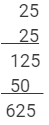This matches with the result obtained by the column method.

(ii) Here, a = 3, b = 7
Step 1. Make 3 columns and write the values of a2, 2 x a x b, and b2 in these columns.

 Column I Column II Column III a2 2 x a x b b2 9 42 49

Step 2. Underline the unit digit of b2 (in Column III) and add its tens digit, if any, with 2 x a x b (in Column II).

 Column I Column II Column III a2 2 x a x b b2 9 42+4 49 46

Step 3. Underline the unit digit in Column II and add the number formed by the tens and other digits, if any, with a2 in Column I.

 Column I Column II Column III a2 2 x a x b b2 9 + 4 42 + 4 49 13 46

Step 4. Underline the number in Column I.

 Column I Column II Column III a2 2 x a x b b2 9 + 4 42 + 4 49 13 46

Step 5. Write the underlined digits at the bottom of each column to obtain the square of the given number.
In this case, we have:
372 = 1369
Using multiplication: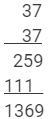This matches with the result obtained using the column method.

(iii) Here, a = 5, b = 4
Step 1. Make 3 columns and write the values of a2, 2 x a x b and b2 in these columns.

 Column I Column II Column III a2 2 x a x b b2 25 40 16

Step 2. Underline the unit digit of b2 (in Column III) and add its tens digit, if any, with 2 x a x b (in Column II).

 Column I Column II Column III a2 2 x a x b b2 25 40 + 1 16 41

Step 3. Underline the unit digit in Column II and add the number formed by the tens and other digits, if any, with a2 in Column I.

 Column I Column II Column III a2 2 x a x b b2 25 + 4 40 + 1 16 29 41

Step 4. Underline the number in Column I.

 Column I Column II Column III a2 2 x a x b b2 25 + 4 40 + 1 16 29 41

Step 5. Write the underlined digits at the bottom of each column to obtain the square of the given number.
In this case, we have:
542 = 2916
Using multiplication: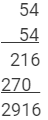This matches with the result obtained using the column method.

(iv) Here, a = 7, b = 1
Step 1. Make 3 columns and write the values of a2, 2 x a x b and b2 in these columns.

 Column I Column II Column III a2 2 x a x b b2 49 14 1

Step 2. Underline the unit digit of b2 (in Column III) and add its tens digit, if any, with 2 x a x b (in Column II).

 Column I Column II Column III a2 2 x a x b b2 49 14 + 0 1 14

Step 3. Underline the unit digit in Column II and add the number formed by the tens and other digits, if any, with a2 in Column I.

 Column I Column II Column III a2 2 x a x b b2 49 + 1 14 + 0 1 50 14

Step 4. Underline the number in Column I.

 Column I Column II Column III a2 2 x a x b b2 49 + 1 14 + 0 1 50 14

Step 5. Write the underlined digits at the bottom of each column to obtain the square of the given number.
In this case, we have:
712 = 5041
Using multiplication: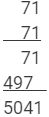This matches with the result obtained using the column method.

(v) Here, a = 9, b = 6
Step 1. Make 3 columns and write the values of a2, 2 x a x b and b2 in these columns.

 Column I Column II Column III a2 2 x a x b b2 81 108 36

Step 2. Underline the unit digit of b2 (in Column III) and add its tens digit, if any, with 2 x a x b (in Column II).

 Column I Column II Column III a2 2 x a x b b2 81 108 + 3 36 111

Step 3. Underline the unit digit in Column II and add the number formed by the tens and other digits, if any, with a2 in Column I.

 Column I Column II Column III a2 2 x a x b b2 81 + 11 108 + 3 36 92 111

Step 4. Underline the number in Column I.

 Column I Column II Column III a2 2 x a x b b2 81 + 11 108 + 3 36 92 111

Step 5. Write the underlined digits at the bottom of each column to obtain the square of the given number.
In this case, we have:
962 = 9216
Using multiplication: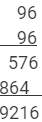This matches with the result obtained using the column method.

####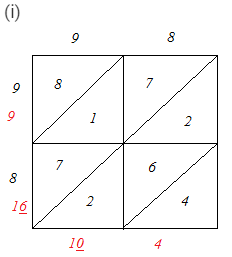∴ 982 = 9604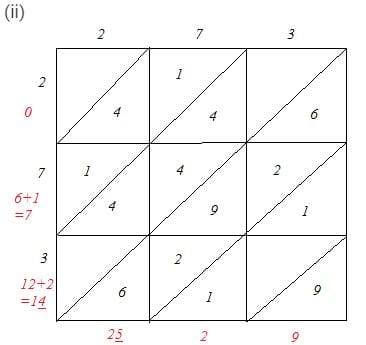∴ 2732 = 74529

(iii)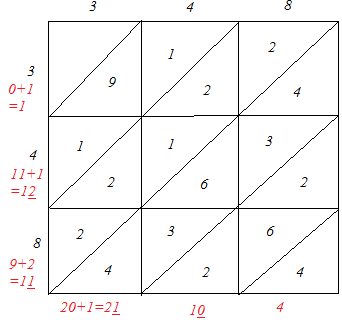∴ 3482 = 121104

(iv)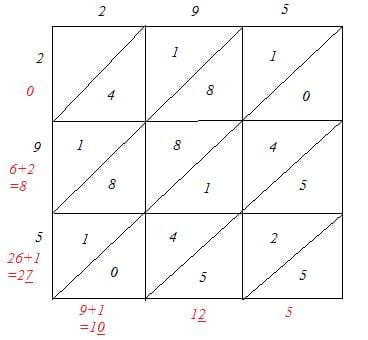∴ 2952 = 87025

(v)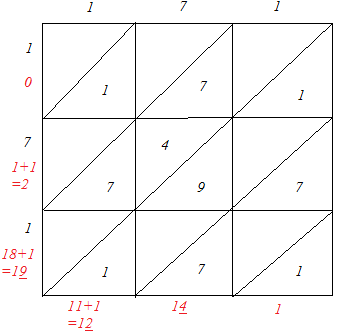∴ 1712 = 29241

#### QUESTION 3: FIND THE SQUARES OF THE FOLLOWING NUMBERS:(I) 127(II) 503(III) 451(IV) 862(V) 265ANSWER 3: We will use visual method as it is the most efficient method to solve this problem.(i) We have:127 = 120 + 7Hence, let us draw a square having side 127 units. Let us split it into 120 units and 7 units.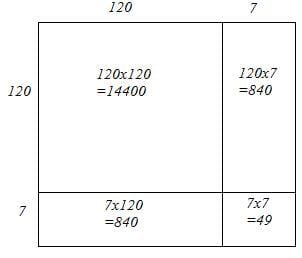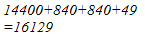Hence, the square of 127 is 16129.

(ii) We have:
503 = 500 + 3
Hence, let us draw a square having side 503 units. Let us split it into 500 units and 3 units.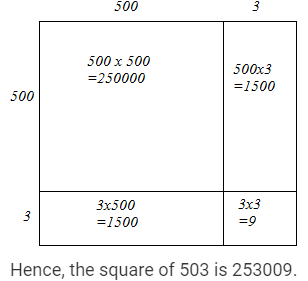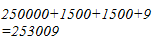Hence, the square of 503 is 253009.

(iii) We have:
451 = 450 + 1
Hence, let us draw a square having side 451 units. Let us split it into 450 units and 1 units.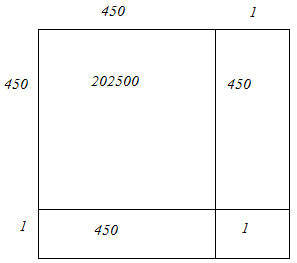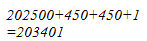Hence, the square of 451 is 203401.

(iv) We have:
862 = 860 + 2
Hence, let us draw a square having side 862 units. Let us split it into 860 units and 2 units.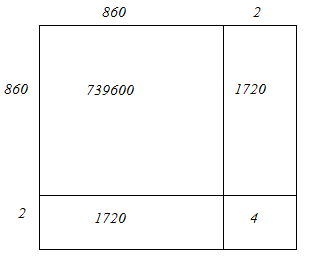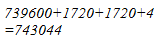Hence, the square of 862 is 743044.

(v) We have:
265 = 260 + 5
Hence, let us draw a square having side 265 units. Let us split it into 260 units and 5 units.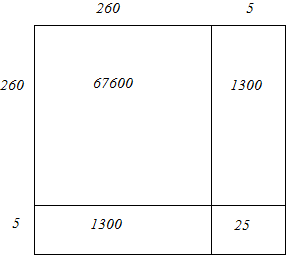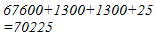Hence, the square of 265 is 70225.

#### Question 4: Find the squares of the following numbers:(i) 425(ii) 575(iii) 405(iv) 205(v) 95(vi) 745(vii) 512(viii) 995Answer 4: Notice that all numbers except the one in question (vii) has 5 as their respective unit digits. We know that the square of a number with the form n5 is a number ending with 25 and has the number n(n + 1) before 25. Notice that all numbers except the one in question (vii) has 5 as their respective unit digits. We know that the square of a number with the form n5 is a number ending with 25 and has the number n(n + 1) before 25.

(i) Here, n = 42
n(+ 1) = (42)(43) = 1806
4252 = 180625
(ii) Here, n = 57
n(+ 1) = (57)(58) = 3306
5752 = 330625
(iii) Here n = 40
n(+ 1) = (40)(41) = 1640
4052 = 164025
(iv) Here n = 20
n(+ 1) = (20)(21) = 420
2052 =  42025
(v) Here n = 9
n(+ 1) = (9)(10) = 90
952 = 9025
(vi) Here n = 74
n(+ 1) = (74)(75) = 5550
7452 = 555025
(vii) We know:
The square of a three-digit number of the form 5ab = (250 + ab)1000 + (ab)2
5122 = (250+12)1000 + (12)2 = 262000 + 144 = 262144
(viii) Here, n = 99
n(+ 1) = (99)(100) = 9900
∴ 9952 = 990025

#### Question 7: Find the squares of the following numbers by visual method:(i) 52(ii) 95(iii) 505(iv) 702(v) 99Answer 7: (i) We have:52 = 50 + 2Let us draw a square having side 52 units. Let us split it into 50 units and 2 units.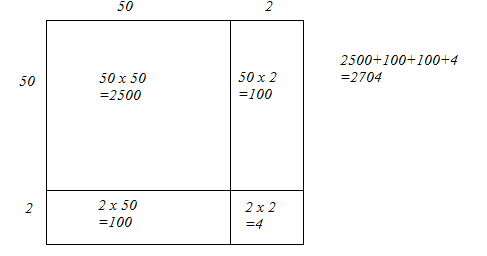The sum of the areas of these four parts is the square of 52. Thus, the square of 52 is 2704.
(ii) We have:
95 = 90 + 5
Let us draw a square having side 95 units. Let us split it into 90 units and 5 units.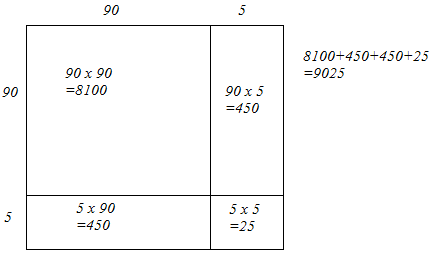The sum of the areas of these four parts is the square of 95. Thus, the square of 95 is 9025.
(iii) We have:
505 = 500 + 5
Let us draw a square having side 505 units. Let us split it into 500 units and 5 units.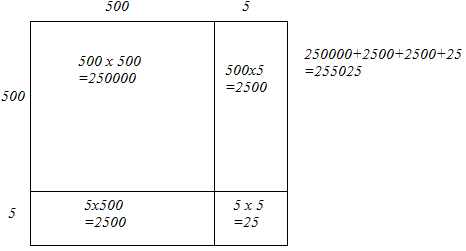The sum of the areas of these four parts is the square of 505. Thus, the square of 505 is 255025.

(iv) We have:
702 = 700 + 2
Let us draw a square having side 702 units. Let us split it into 700 units and 2 units.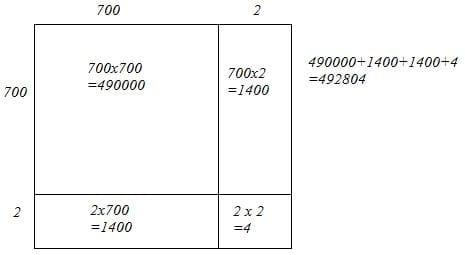The sum of the areas of these four parts is the square of 702. Thus, the square of 702 is 492804.

(v) We have:
99 = 90 + 9
Let us draw a square having side 99 units. Let us split it into 90 units and 9 units.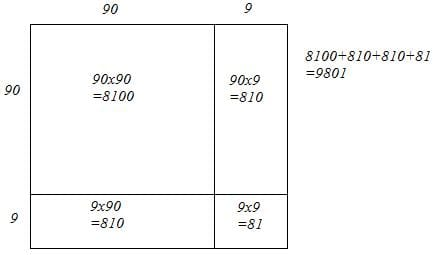The sum of the areas of these four parts is the square of 99. Thus, the square of 99 is 9801.

The document RD Sharma Solutions - Chapter 3 - Squares and Square Roots (Ex-3.3) - Class 8 Math Notes | Study RD Sharma Solutions for Class 8 Mathematics - Class 8 is a part of the Class 8 Course RD Sharma Solutions for Class 8 Mathematics.
All you need of Class 8 at this link: Class 8Use Code STAYHOME200 and get INR 200 additional OFF

## RD Sharma Solutions for Class 8 Mathematics

88 docs

Track your progress, build streaks, highlight & save important lessons and more!

,

,

,

,

,

,

,

,

,

,

,

,

,

,

,

,

,

,

,

,

,

;